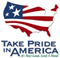## Conversion factors, water-quality units, and vertical datums

This report uses English and metric units. To determine equivalent metric values from English values, multiply the English values by the conversion factors listed below. To determine equivalent English values from metric values, divide the metric values by the conversion factors listed below.

Multiply By To Obtain
Length
inch (in.) 25.4 millimeter (mm)
inch (in.) 2.54 centimeter (cm)
foot (ft) 0.3048 meter (m)
mile (mi) 1.609 kilometer (km)
Area
acre 4,047 square meter (m2)
square foot (ft2) 0.09290 square meter (m2)
square mile (mi2) 2.590 square kilometer (km2)
Volume
gallon (gal) 3.785 liter (L)
cubic foot (ft3) 0.02832 cubic meter (m3)
Flow
inch per year (in/yr) 25.4 millimeter per year (mm/yr)
foot per year (ft/yr) 0.3048 meter per year (m/yr)
cubic foot per second (ft3/s) 0.02832 cubic meter per second (m3/s)
gallon per minute (gal/min) 0.06308 liter per second (L/s)
million gallons per day (Mgal/d) 0.04381 cubic meter per second (m3/s)
billion gallons per day (Bgal/d) 43.81 cubic meter per second (m3/s)

Temperature is given in degree Celsius (°C), which can be converted to degree Fahrenheit (°F) by the following equation: °F = 1.8 (°C) + 32

#### Water-Quality Units

Abbreviations:
grams per cubic centimeter (g/cm3)
milligrams per liter (mg/L)
parts per million (ppm)
parts per thousand (ppt)

Conversions: Most chemical concentrations in this report are given in milligrams per liter, which is a unit expressing the concentration of chemical constituents in solution as weight (milligrams) of solute per unit volume (liter) of water. A few of the chemical concentrations are given as parts per thousand or parts per million; these are units of weight of solute per weight of water. Parts per thousand (that is, grams of solute per kilogram of water) is a concentration that is often used in reporting the composition of seawater. Concentration expressed as parts per million (that is, milligrams of solute per kilogram of water) can be converted to milligrams per liter by multiplying the concentration by the density of water, in kilograms per liter. At low concentrations, such as that of freshwater, concentrations expressed as parts per million are nearly equal to those expressed as milligrams per liter.

#### Vertical Datums

Because this report is based on a large number of previously published scientific investigations, "sea level" is not referenced to a single vertical datum. "Mean sea level" also is not used with reference to a single datum; where used, the phrase means the average surface of the ocean as determined by calibration of measurements at tidal stations. The vertical datum used for each investigation described in this report is identified where it could be determined from the published sources of information.

 AccessibilityFOIAPrivacyPolicies and Notices U.S. Department of the Interior, U.S. Geological Survey Persistent URL: Page Contact Information: Contact USGS Last modified: Wednesday, November 23 2016, 12:23:12 PM